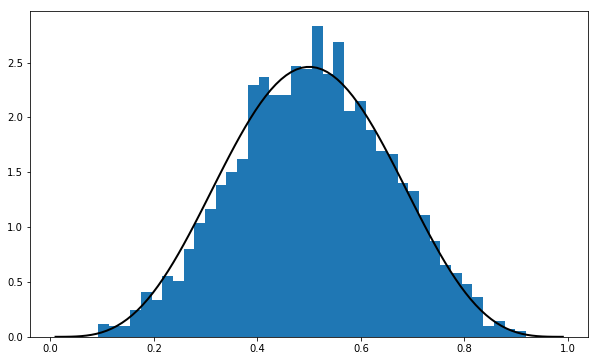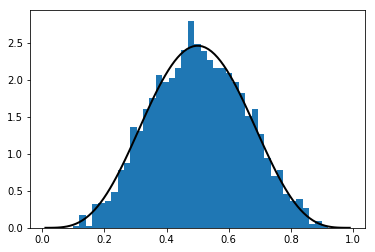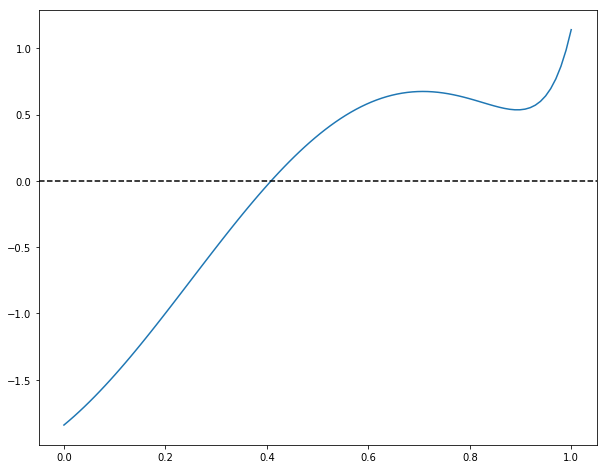## Download PDF

How to read this lecture...

Code should execute sequentially if run in a Jupyter notebook

# SciPy¶

## Contents¶

SciPy builds on top of NumPy to provide common tools for scientific programming such as

Like NumPy, SciPy is stable, mature and widely used.

Many SciPy routines are thin wrappers around industry-standard Fortran libraries such as LAPACK, BLAS, etc.

It’s not really necessary to “learn” SciPy as a whole.

A more common approach is to get some idea of what’s in the library and then look up documentation as required.

In this lecture, we aim only to highlight some useful parts of the package.

## SciPy versus NumPy¶

SciPy is a package that contains various tools that are built on top of NumPy, using its array data type and related functionality.

In fact, when we import SciPy we also get NumPy, as can be seen from the SciPy initialization file

In :
# Import numpy symbols to scipy namespace
import numpy as _num
linalg = None
from numpy import *
from numpy.random import rand, randn
from numpy.fft import fft, ifft
from numpy.lib.scimath import *

__all__  = []
__all__ += _num.__all__
__all__ += ['randn', 'rand', 'fft', 'ifft']

del _num
# Remove the linalg imported from numpy so that the scipy.linalg package can be
# imported.
del linalg
__all__.remove('linalg')


However, it’s more common and better practice to use NumPy functionality explicitly

In :
import numpy as np

a = np.identity(3)


What is useful in SciPy is the functionality in its sub-packages

• scipy.optimize, scipy.integrate, scipy.stats, etc.

These sub-packages and their attributes need to be imported separately

In :
from scipy.integrate import quad
from scipy.optimize import brentq
# etc


Let’s explore some of the major sub-packages.

## Statistics¶

The scipy.stats subpackage supplies

• numerous random variable objects (densities, cumulative distributions, random sampling, etc.)
• some estimation procedures
• some statistical tests

### Random Variables and Distributions¶

Recall that numpy.random provides functions for generating random variables

In :
np.random.beta(5, 5, size=3)

Out:
array([0.36000421, 0.54578195, 0.50458823])

This generates a draw from the distribution below when a, b = 5, 5

$$f(x; a, b) = \frac{x^{(a - 1)} (1 - x)^{(b - 1)}} {\int_0^1 u^{(a - 1)} (1 - u)^{(b - 1)} du} \qquad (0 \leq x \leq 1) \tag{1}$$

Sometimes we need access to the density itself, or the cdf, the quantiles, etc.

For this, we can use scipy.stats, which provides all of this functionality as well as random number generation in a single consistent interface.

Here’s an example of usage

In :
from scipy.stats import beta
import matplotlib.pyplot as plt
%matplotlib inline

q = beta(5, 5)      # Beta(a, b), with a = b = 5
obs = q.rvs(2000)   # 2000 observations
grid = np.linspace(0.01, 0.99, 100)

fig, ax = plt.subplots(figsize=(10, 6))
ax.hist(obs, bins=40, density=True)
ax.plot(grid, q.pdf(grid), 'k-', linewidth=2)
plt.show()In this code, we created a so-called rv_frozen object, via the call q = beta(5, 5).

The “frozen” part of the notation implies that q represents a particular distribution with a particular set of parameters.

Once we’ve done so, we can then generate random numbers, evaluate the density, etc., all from this fixed distribution

In :
q.cdf(0.4)      # Cumulative distribution function

Out:
0.26656768000000003
In :
q.pdf(0.4)      # Density function

Out:
2.0901888000000013
In :
q.ppf(0.8)      # Quantile (inverse cdf) function

Out:
0.6339134834642708
In :
q.mean()

Out:
0.5

The general syntax for creating these objects is

identifier = scipy.stats.distribution_name(shape_parameters)

where distribution_name is one of the distribution names in scipy.stats.

There are also two keyword arguments, loc and scale, which following our example above, are called as

identifier = scipy.stats.distribution_name(shape_parameters, loc=c, scale=d)

These transform the original random variable $X$ into $Y = c + d X$.

The methods rvs, pdf, cdf, etc. are transformed accordingly.

Before finishing this section, we note that there is an alternative way of calling the methods described above.

For example, the previous code can be replaced by

In :
obs = beta.rvs(5, 5, size=2000)
grid = np.linspace(0.01, 0.99, 100)

fig, ax = plt.subplots()
ax.hist(obs, bins=40, density=True)
ax.plot(grid, beta.pdf(grid, 5, 5), 'k-', linewidth=2)
plt.show()### Other Goodies in scipy.stats¶

There are a variety statistical functions in scipy.stats.

For example, scipy.stats.linregress implements simple linear regression

In :
from scipy.stats import linregress

x = np.random.randn(200)
y = 2 * x + 0.1 * np.random.randn(200)
gradient, intercept, r_value, p_value, std_err = linregress(x, y)
gradient, intercept

Out:
(2.001513549791738, 0.00954386798303633)

To see the full list, consult the documentation.

## Roots and Fixed Points¶

A root of a real function $f$ on $[a,b]$ is an $x \in [a, b]$ such that $f(x)=0$.

For example, if we plot the function

$$f(x) = \sin(4 (x - 1/4)) + x + x^{20} - 1 \tag{2}$$

with $x \in [0,1]$ we get

In :
f = lambda x: np.sin(4 * (x - 1/4)) + x + x**20 - 1
x = np.linspace(0, 1, 100)

fig, ax = plt.subplots(figsize=(10, 8))
ax.plot(x, f(x))
ax.axhline(ls='--', c='k')
plt.show()The unique root is approximately 0.408.

Let’s consider some numerical techniques for finding roots.

### Bisection¶

One of the most common algorithms for numerical root-finding is bisection.

To understand the idea, recall the well-known game where

• Player A thinks of a secret number between 1 and 100
• Player B asks if it’s less than 50

• If yes, B asks if it’s less than 25
• If no, B asks if it’s less than 75

And so on.

This is bisection.

Here’s a fairly simplistic implementation of the algorithm in Python.

It works for all sufficiently well behaved increasing continuous functions with $f(a) < 0 < f(b)$

In :
def bisect(f, a, b, tol=10e-5):
"""
Implements the bisection root finding algorithm, assuming that f is a
real-valued function on [a, b] satisfying f(a) < 0 < f(b).
"""
lower, upper = a, b

while upper - lower > tol:
middle = 0.5 * (upper + lower)
# === if root is between lower and middle === #
if f(middle) > 0:
lower, upper = lower, middle
# === if root is between middle and upper  === #
else:
lower, upper = middle, upper

return 0.5 * (upper + lower)


In fact, SciPy provides its own bisection function, which we now test using the function $f$ defined in (2)

In :
from scipy.optimize import bisect

bisect(f, 0, 1)

Out:
0.4082935042806639

### The Newton-Raphson Method¶

Another very common root-finding algorithm is the Newton-Raphson method.

In SciPy this algorithm is implemented by scipy.optimize.newton.

Unlike bisection, the Newton-Raphson method uses local slope information.

This is a double-edged sword:

• When the function is well-behaved, the Newton-Raphson method is faster than bisection.
• When the function is less well-behaved, the Newton-Raphson might fail.

Let’s investigate this using the same function $f$, first looking at potential instability

In :
from scipy.optimize import newton

newton(f, 0.2)   # Start the search at initial condition x = 0.2

Out:
0.40829350427935673
In :
newton(f, 0.7)   # Start the search at x = 0.7 instead

Out:
0.7001700000000279

The second initial condition leads to failure of convergence.

On the other hand, using IPython’s timeit magic, we see that newton can be much faster

In :
%timeit bisect(f, 0, 1)

68.7 µs ± 309 ns per loop (mean ± std. dev. of 7 runs, 10000 loops each)

In :
%timeit newton(f, 0.2)

147 µs ± 118 ns per loop (mean ± std. dev. of 7 runs, 10000 loops each)


### Hybrid Methods¶

So far we have seen that the Newton-Raphson method is fast but not robust.

This bisection algorithm is robust but relatively slow.

This illustrates a general principle

• If you have specific knowledge about your function, you might be able to exploit it to generate efficiency.
• If not, then the algorithm choice involves a trade-off between the speed of convergence and robustness.

In practice, most default algorithms for root-finding, optimization and fixed points use hybrid methods.

These methods typically combine a fast method with a robust method in the following manner:

1. Attempt to use a fast method
2. Check diagnostics
3. If diagnostics are bad, then switch to a more robust algorithm

In scipy.optimize, the function brentq is such a hybrid method and a good default

In :
brentq(f, 0, 1)

Out:
0.40829350427936706
In :
%timeit brentq(f, 0, 1)

17.6 µs ± 19.8 ns per loop (mean ± std. dev. of 7 runs, 100000 loops each)


Here the correct solution is found and the speed is almost the same as newton.

### Multivariate Root-Finding¶

Use scipy.optimize.fsolve, a wrapper for a hybrid method in MINPACK.

See the documentation for details.

### Fixed Points¶

SciPy has a function for finding (scalar) fixed points too

In :
from scipy.optimize import fixed_point

fixed_point(lambda x: x**2, 10.0)  # 10.0 is an initial guess

Out:
array(1.)

If you don’t get good results, you can always switch back to the brentq root finder, since the fixed point of a function $f$ is the root of $g(x) := x - f(x)$.

## Optimization¶

Most numerical packages provide only functions for minimization.

Maximization can be performed by recalling that the maximizer of a function $f$ on domain $D$ is the minimizer of $-f$ on $D$.

Minimization is closely related to root-finding: For smooth functions, interior optima correspond to roots of the first derivative.

The speed/robustness trade-off described above is present with numerical optimization too.

Unless you have some prior information you can exploit, it’s usually best to use hybrid methods.

For constrained, univariate (i.e., scalar) minimization, a good hybrid option is fminbound

In :
from scipy.optimize import fminbound

fminbound(lambda x: x**2, -1, 2)  # Search in [-1, 2]

Out:
0.0

### Multivariate Optimization¶

Multivariate local optimizers include minimize, fmin, fmin_powell, fmin_cg, fmin_bfgs, and fmin_ncg.

Constrained multivariate local optimizers include fmin_l_bfgs_b, fmin_tnc, fmin_cobyla.

See the documentation for details.

## Integration¶

Most numerical integration methods work by computing the integral of an approximating polynomial.

The resulting error depends on how well the polynomial fits the integrand, which in turn depends on how “regular” the integrand is.

In SciPy, the relevant module for numerical integration is scipy.integrate.

A good default for univariate integration is quad

In :
from scipy.integrate import quad

integral, error = quad(lambda x: x**2, 0, 1)
integral

Out:
0.33333333333333337

In fact, quad is an interface to a very standard numerical integration routine in the Fortran library QUADPACK.

It uses Clenshaw-Curtis quadrature, based on expansion in terms of Chebychev polynomials.

There are other options for univariate integration—a useful one is fixed_quad, which is fast and hence works well inside for loops.

There are also functions for multivariate integration.

See the documentation for more details.

## Linear Algebra¶

We saw that NumPy provides a module for linear algebra called linalg.

SciPy also provides a module for linear algebra with the same name.

The latter is not an exact superset of the former, but overall it has more functionality.

We leave you to investigate the set of available routines.

## Exercises¶

### Exercise 1¶

Previously we discussed the concept of recursive function calls.

Write a recursive implementation of the bisection function described above, which we repeat here for convenience.

In :
def bisect(f, a, b, tol=10e-5):
"""
Implements the bisection root finding algorithm, assuming that f is a
real-valued function on [a, b] satisfying f(a) < 0 < f(b).
"""
lower, upper = a, b

while upper - lower > tol:
middle = 0.5 * (upper + lower)
# === if root is between lower and middle === #
if f(middle) > 0:
lower, upper = lower, middle
# === if root is between middle and upper  === #
else:
lower, upper = middle, upper

return 0.5 * (upper + lower)


Test it on the function f = lambda x: np.sin(4 * (x - 0.25)) + x + x**20 - 1 discussed above.

## Solutions¶

### Exercise 1¶

Here’s a reasonable solution:

In :
def bisect(f, a, b, tol=10e-5):
"""
Implements the bisection root-finding algorithm, assuming that f is a
real-valued function on [a, b] satisfying f(a) < 0 < f(b).
"""
lower, upper = a, b
if upper - lower < tol:
return 0.5 * (upper + lower)
else:
middle = 0.5 * (upper + lower)
print(f'Current mid point = {middle}')
if f(middle) > 0:   # Implies root is between lower and middle
return bisect(f, lower, middle)
else:               # Implies root is between middle and upper
return bisect(f, middle, upper)


We can test it as follows

In :
f = lambda x: np.sin(4 * (x - 0.25)) + x + x**20 - 1
bisect(f, 0, 1)

Current mid point = 0.5
Current mid point = 0.25
Current mid point = 0.375
Current mid point = 0.4375
Current mid point = 0.40625
Current mid point = 0.421875
Current mid point = 0.4140625
Current mid point = 0.41015625
Current mid point = 0.408203125
Current mid point = 0.4091796875
Current mid point = 0.40869140625
Current mid point = 0.408447265625
Current mid point = 0.4083251953125
Current mid point = 0.40826416015625

Out:
0.408294677734375
• Share page